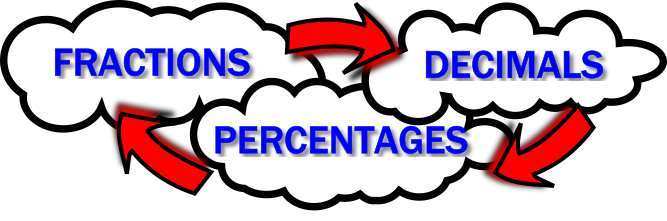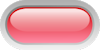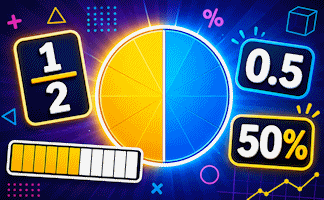#Fraction Decimal Percentage$$\frac12$$ $$0.5$$ $$50$$%$$\frac34$$ $$0.75$$ $$75$$%$$\frac14$$ $$0.25$$ $$25$$%$$\frac15$$ $$0.2$$ $$20$$%$$\frac35$$ $$0.6$$ $$60$$%$$\frac{11}{20}$$ $$0.55$$ $$55$$%$$\frac{7}{10}$$ $$0.7$$ $$70$$%$$\frac{1}{25}$$ $$0.04$$ $$4$$%$$\frac{3}{10}$$ $$0.3$$ $$30$$%$$\frac{3}{100}$$ $$0.03$$ $$3$$%$$\frac{17}{20}$$ $$0.85$$ $$85$$%$$\frac{1}{3}$$ $$0.\dot3$$ $$33\frac13$$%$$\frac{2}{3}$$ $$0.\dot6$$ $$66\frac23$$%$$\frac{1}{8}$$ $$0.125$$ $$12\frac12$$%$$\frac{3}{8}$$ $$0.375$$ $$37\frac12$$%$$\frac{7}{8}$$ $$0.875$$ $$87\frac12$$%$$\frac{5}{8}$$ $$0.625$$ $$62\frac12$$%$$\frac{1}{40}$$ $$0.025$$ $$2\frac12$$%$$\frac{17}{40}$$ $$0.425$$ $$42\frac12$$%$$\frac{7}{200}$$ $$0.035$$ $$3\frac12$$%$$\frac{7}{150}$$ $$0.04\dot6$$ $$4\frac23$$%## Copy and complete this table.

Teacher! You can customise this starter to suit your pupils. Click here to show all possible rows in the table above then click on the red buttons to hide the rows you don't want.

A short slide presentation is available to revise the operations needed to convert between fractions, decimals and percentages.

## A Mathematics Lesson Starter Of The Day

Share

How did you use this starter? Can you suggest how teachers could present or develop this resource? Do you have any comments? It is always useful to receive feedback and helps make this free resource even more useful for Maths teachers anywhere in the world.

If you don't have the time to provide feedback we'd really appreciate it if you could give this page a score! We are constantly improving and adding to these starters so it would be really helpful to know which ones are most useful. Simply click on a button below:

Excellent, I would like to see more like this
Good, achieved the results I required
Satisfactory
Didn't really capture the interest of the students
Not for me! I wouldn't use this type of activity.

This starter has scored a mean of 2.9 out of 5 based on 342 votes.

Previous Day | This starter is for 27 July | Next Day

Your access to the majority of the Transum resources continues to be free but you can help support the continued growth of the website by doing your Amazon shopping using the links on this page. Below is an Amazon search box and some items chosen and recommended by Transum Mathematics to get you started.

## GCSE Revision and Practice

Whatever exam board you use for GCSE Mathematics, this book by David Rayner remains an all-round winner. With this latest edition presented in full colour and completely updated for the new GCSE(9-1) specifications, this uniquely effective text continues to increase your chance of obtaining a good grade.

This book is targeted at the Higher tier GCSE, and provides a wealth of practice with careful progression, alongside substantial revision support for the new-style grading and exam questions. With all the new topics included, and a dedicated section on using and applying mathematics, this unique resource can be used either as a course book over two or three years or as a revision text in the run-up to exams. more...Teacher, do your students have access to computers?Do they have iPads or Laptops in Lessons? Whether your students each have a TabletPC, a Surface or a Mac, this activity lends itself to eLearning (Engaged Learning).Transum.org/go/?Start=July27

Here is the URL which will take them to a related student activity.

Transum.org/go/?to=fdp## Curriculum Reference

See the National Curriculum page for links to related online activities and resources.For All: